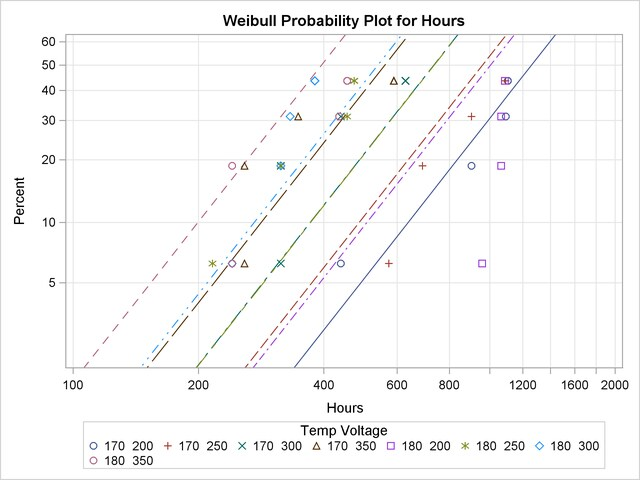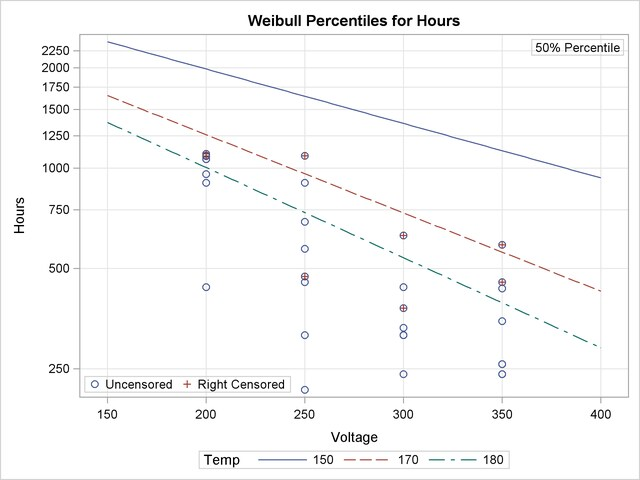The RELIABILITY Procedure
 Regression Model with Two Independent Variables

Meeker and Escobar (1998, p. 447) analyzed data from an accelerated test on the lifetimes of glass capacitors as a function of operating voltage and temperature. The following SAS statements create a SAS data set containing the data. There are four lifetimes for each of eight combinations and four censored observations after the fourth failure for each combination:

```data glass;
input Temp Voltage @;
do i = 1 to 4;
cen = 0;
input Hours @; output;
end;
do i = 1 to 4;
cen = 1;
output;
end;
datalines;
170 200  439  904  1092  1105
170 250  572  690   904  1090
170 300  315  315   439   628
170 350  258  258   347   588
180 200  959 1065  1065  1087
180 250  216  315   455   473
180 300  241  315   332   380
180 350  241  241   435   455
;
run;
```

The following statements analyze the capacitor data. The MODEL statement fits a regression model with Temp and Voltage as independent variables. Parameter estimates from the fitted regression model are shown in Figure 12.24. An interaction term between Temp and Voltage is included. The PPLOT statement creates a Weibull probability plot shown in Figure 12.25 with all temperature-voltage combinations overlaid on the same plot. The regression model fit is also plotted. The RPLOT statement creates the plot shown in Figure 12.26 of the data and Weibull distribution percentiles from the regression model as a function of voltage for values of temperature of 150, 170, and 180:

```proc reliability data = glass;
distribution Weibull;
model Hours*cen(1) = temp voltage temp * voltage;
pplot Hours*cen(1) = ( temp voltage ) / fit = model
overlay
noconf
lupper = 2000;
run;
```
```proc reliability data = glass;
distribution Weibull;
model Hours*cen(1) = temp voltage temp * voltage;
rplot Hours*cen(1) = voltage / fit = regression(temp = 150, 170, 180)
plotfit;
run;
```

Figure 12.24 Parameter Estimates for Fitted Regression Model
The RELIABILITY Procedure

Weibull Parameter Estimates
Parameter Estimate Standard Error Asymptotic Normal
95% Confidence Limits
Lower Upper
Intercept 9.4135 10.5402 -11.2449 30.0719
Temp -0.0062 0.0598 -0.1235 0.1110
Voltage 0.0086 0.0374 -0.0648 0.0820
Temp*Voltage -0.0001 0.0002 -0.0005 0.0003
EV Scale 0.3624 0.0553 0.2687 0.4887
Weibull Shape 2.7593 0.4210 2.0461 3.7209

Figure 12.25 Probability Plot for Glass Capacitor Regression ModelFigure 12.26 Plot of Data and Fitted Weibull Percentiles for Glass Capacitor Regression ModelPrevious Page | Next Page | Top of Page